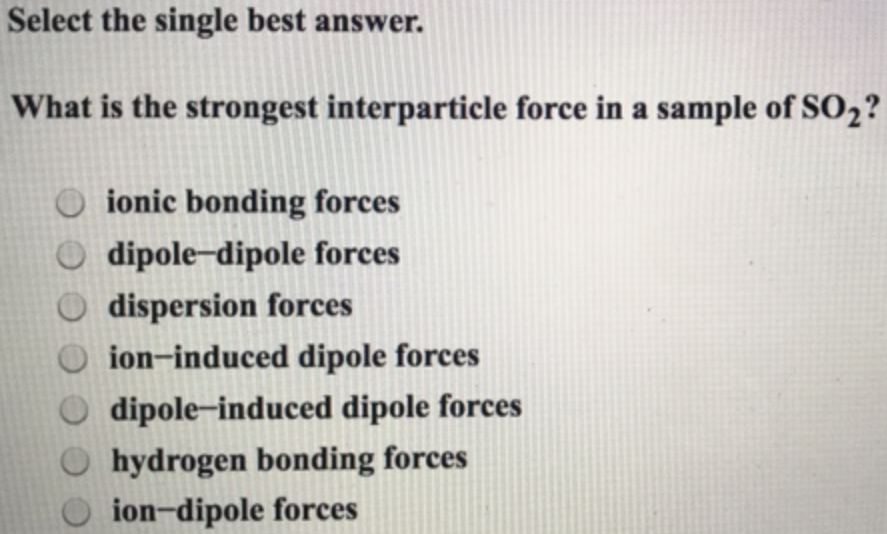# Problem: Select the single best answer. What is the strongest interparticle force in a sample of SO 2? (A) ionic bonding forces (B) dipole-dipole forces (C) dispersion forces (D) ion-induced dipole forces (E) dipole-induced dipole forces (F) hydrogen bonding forces (G) ion-dipole forces

###### FREE Expert Solution
87% (201 ratings)###### Problem Details

What is the strongest interparticle force in a sample of SO 2

(A) ionic bonding forces

(B) dipole-dipole forces

(C) dispersion forces

(D) ion-induced dipole forces

(E) dipole-induced dipole forces

(F) hydrogen bonding forces

(G) ion-dipole forces